Image Segmentation (demo)
The objective is the fast and precise image segmentation.  Some examples are shown below.
Edge Detection (demo)
Canny Edge Detection.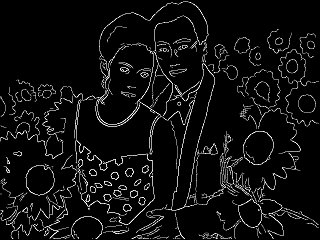Sigma= 1, Low= 0.4, High= 0.8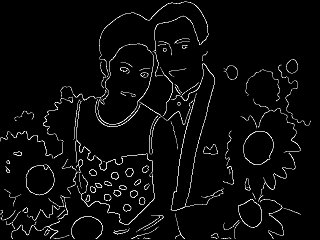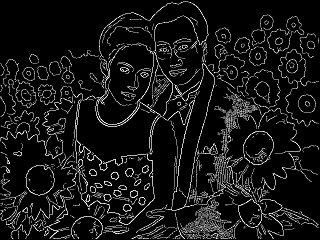Sigma= 2, Low= 0.4, High= 0.8 Sigma= 1, Low= 0.3, High= 0.7
Line and Arc Finding (demo)

To extract the lines and Arcs in the images. (click on the image to see the bigger image showing the detected lines and arcs)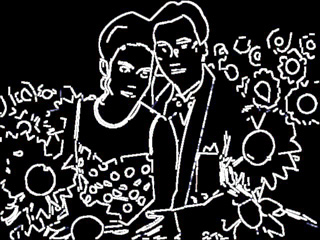Iterative K-Means Clustering (demo)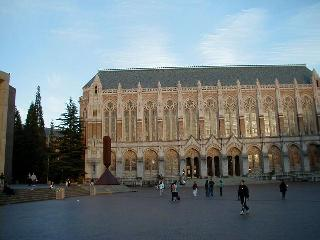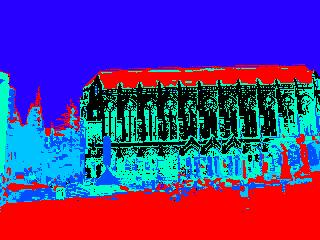Resampling (demo)